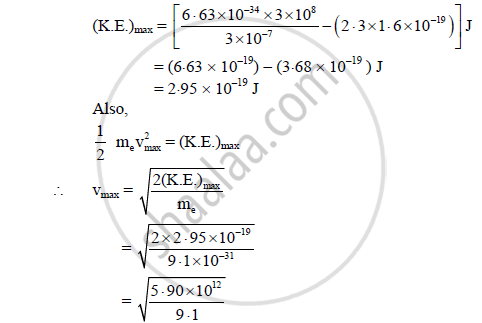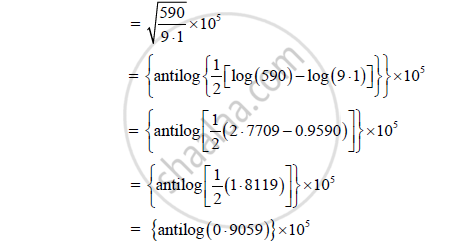HSC Science (Electronics) 12th Board ExamMaharashtra State Board
Share

# Light of Wavelength 3000å Falls on a Metal Surface Having Work Function 2.3 Ev. Calculate the Maximum Velocity of Ejected Electrons - HSC Science (Electronics) 12th Board Exam - Physics

#### Question

Light of wavelength 3000Å falls on a metal surface having work function 2.3 eV. Calculate the maximum velocity of ejected electrons

(Planck's constant h = 6.63 x 10-34 J.s., Velocity of light c = 3 x 108 m/s, mass of an electron = 9.1 x 10-31 kg)

#### Solution

Given: λ = 3000 Å = 3 x 10–7 m, me = 9.1  10–31 kg,

Φo = 2.3 eV, h = 6.63  10–34 Js, c = 3  108 m/s

To find: Maximum velocity (vmax)

Formula: (K.E)max = (hc)/λ - Φ_o

Calculation: From formula,= 8.052 x 105 m/s

The maximum velocity of electron is 8.052 x 105 m/s

Is there an error in this question or solution?

#### APPEARS IN

2015-2016 (July) (with solutions)
Question 7.4 | 3.00 marks

#### Video TutorialsVIEW ALL 

Solution Light of Wavelength 3000å Falls on a Metal Surface Having Work Function 2.3 Ev. Calculate the Maximum Velocity of Ejected Electrons Concept: Wave Theory of Light.
S